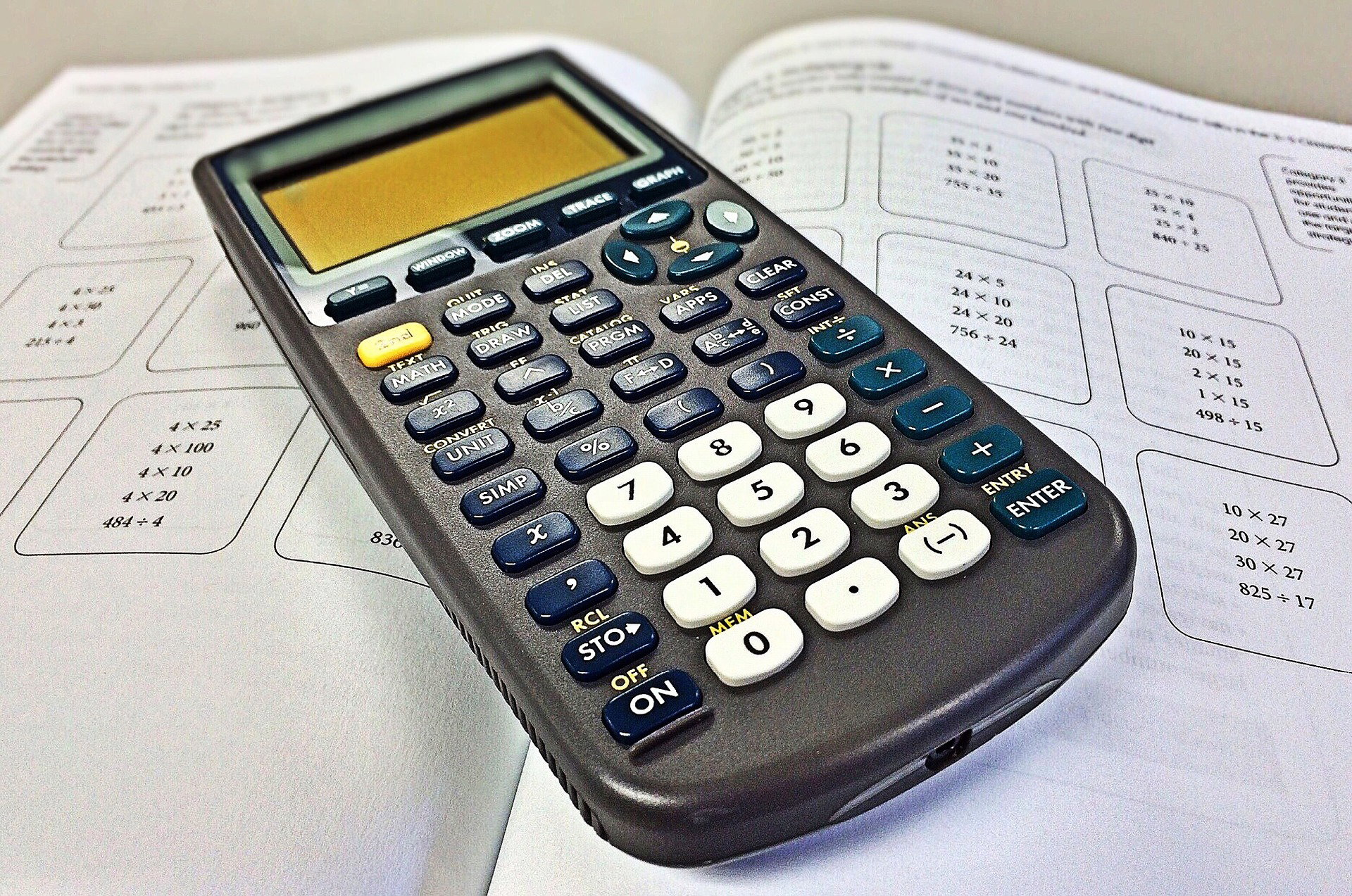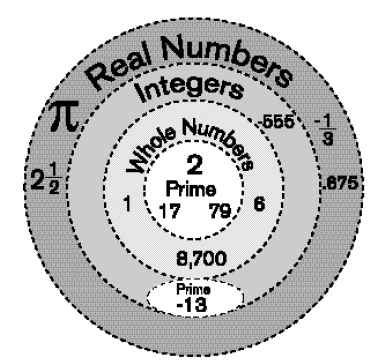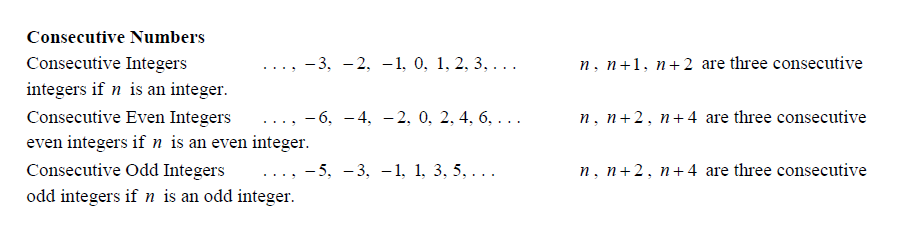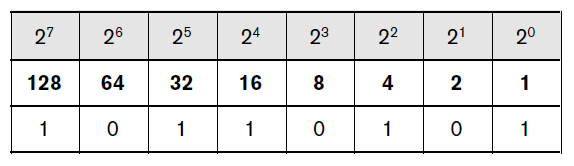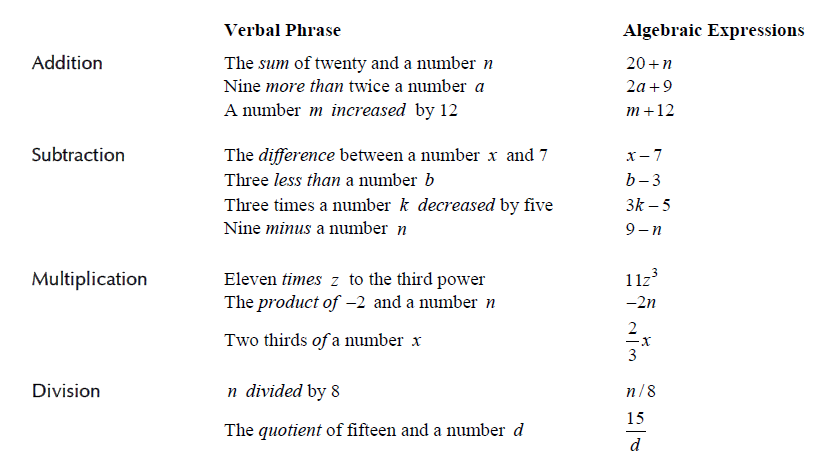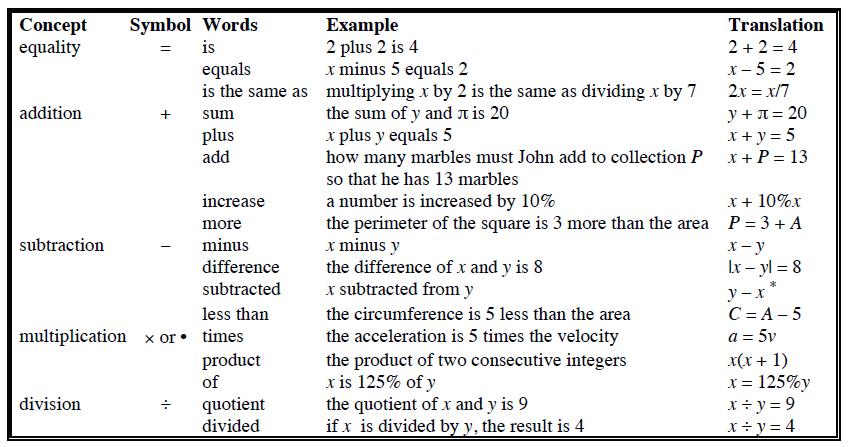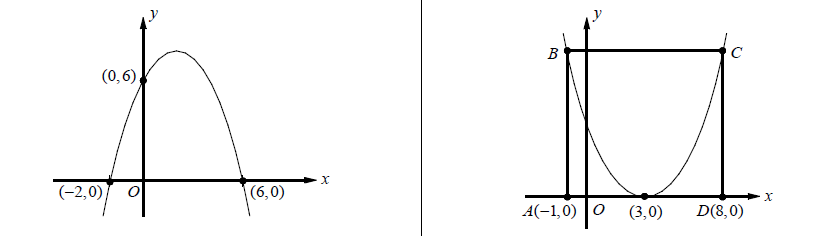In Algebra, a quadratic function is a polynomial function in which the highest-degree term is of the second degree.

The graph of a quadratic function is a curve called a parabola. Parabolas may open upward or downward and vary in "width" or "steepness", but they all have the same basic "U" shape. All parabolas are symmetric with respect to a line called the axis of symmetry. A parabola intersects its axis of symmetry at a point called the vertex of the parabola.

A univariate quadratic function can be expressed in three formats: standard form, factored form and vertex form.  Standard form is f(x)=ax^2+bx+c, where a,b and are coefficients and a cannot be zero. Factored form is f(x)=a(x-r1)(x-r2) where r1and r2 are the roots of the quadratic function and the solutions of the corresponding quadratic equation. Vertex form is f(x)=a(x-h)^2+k, where and are the and coordinates of the vertex, respectively. The coefficient a has the same value in all three forms.

If coefficient a > 0, the parabola opens upwards. If a < 0, the parabola opens downwards. The coefficient controls the degree of curvature of the graph, a larger magnitude of gives the graph a more closed (sharply curved) appearance. The coefficients and together control the location of the vertex (T(h,k)), where h=-b/a and k=(-b^2+4ac)/4a. The coefficient is the height of the parabola where it intercepts the y-axis.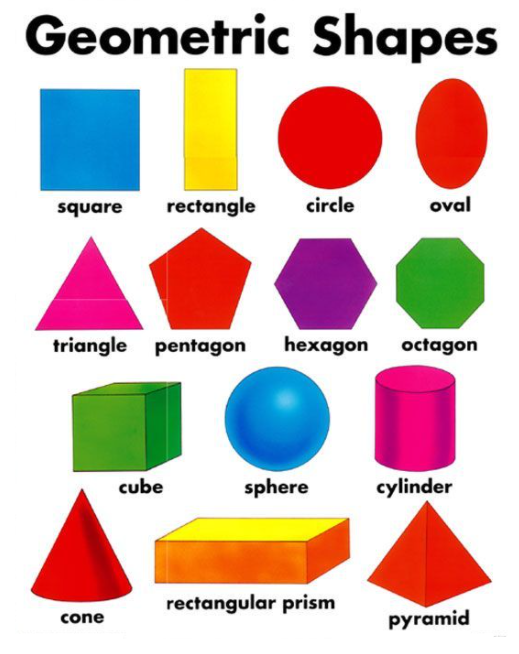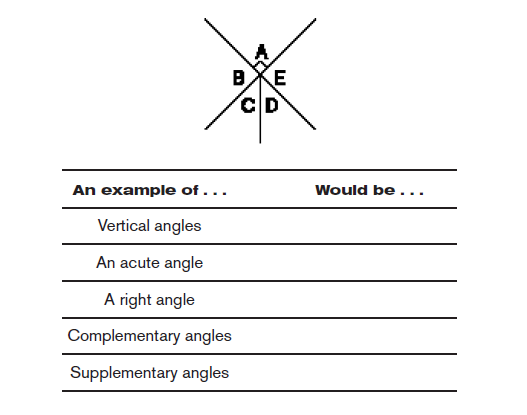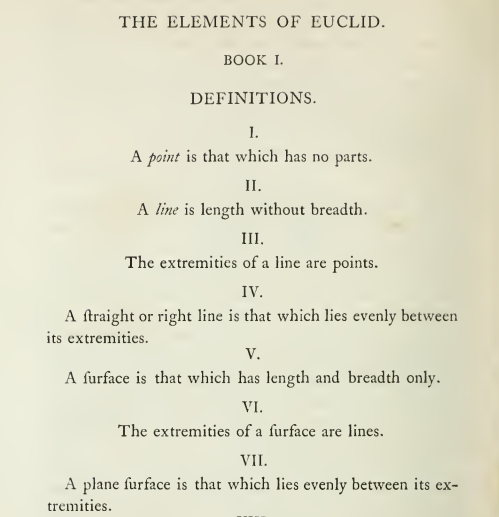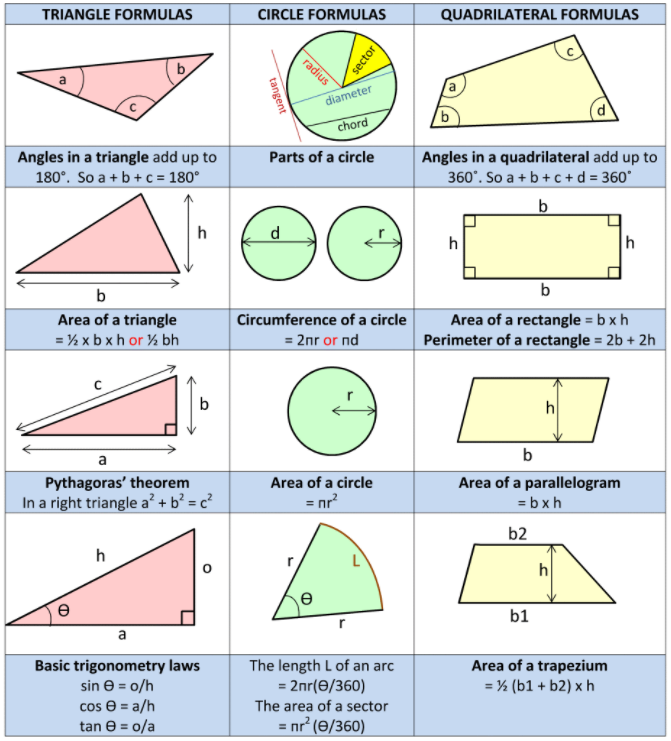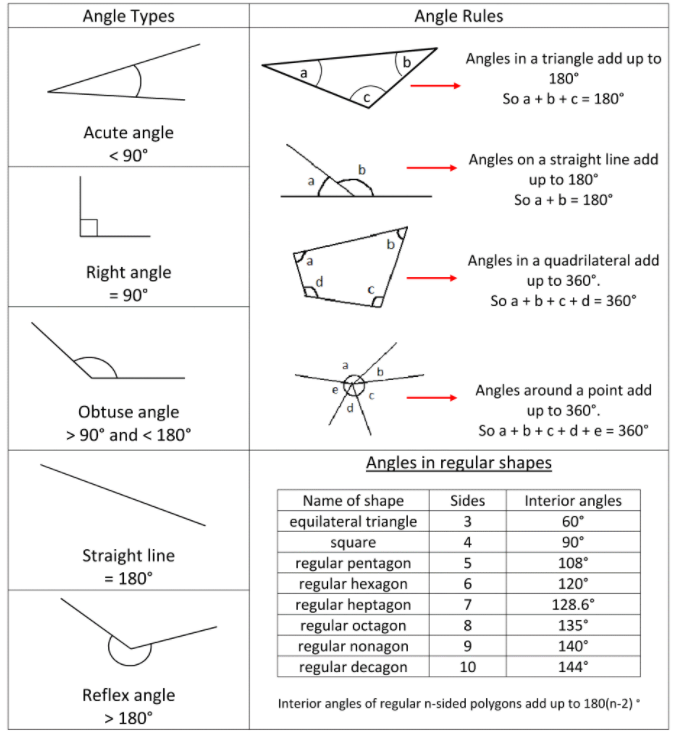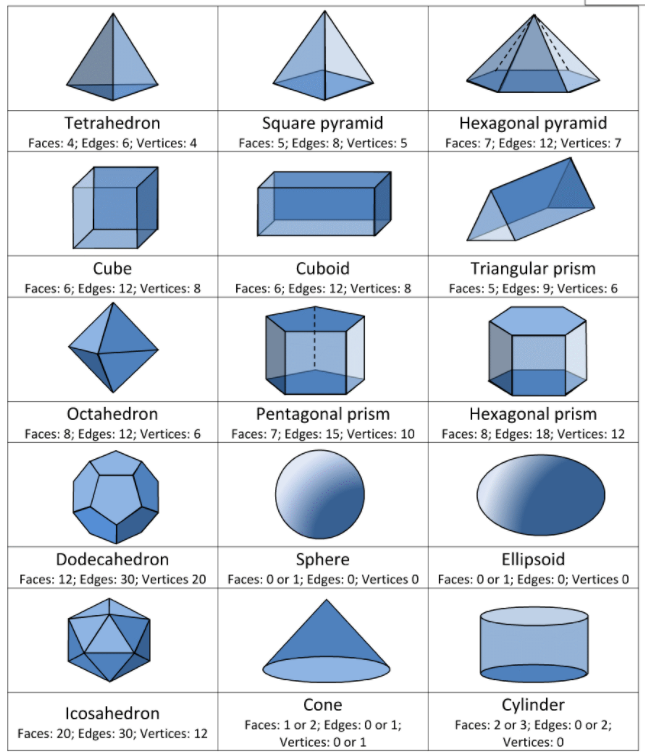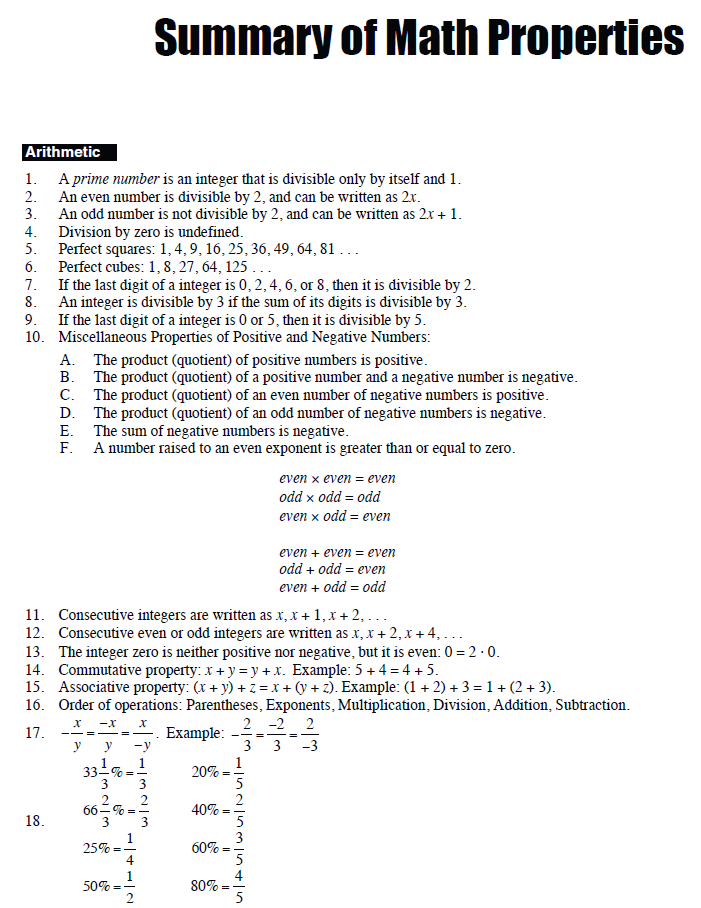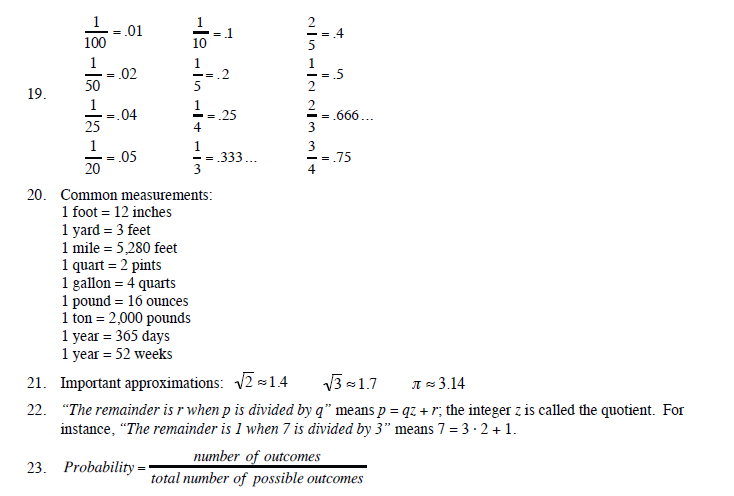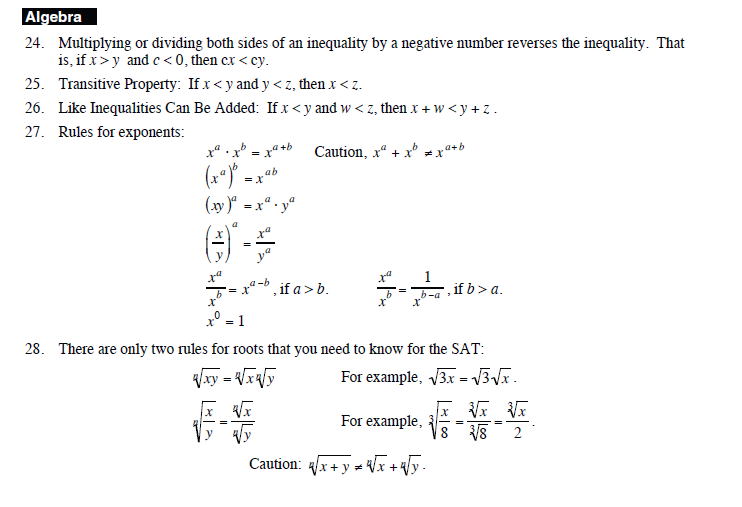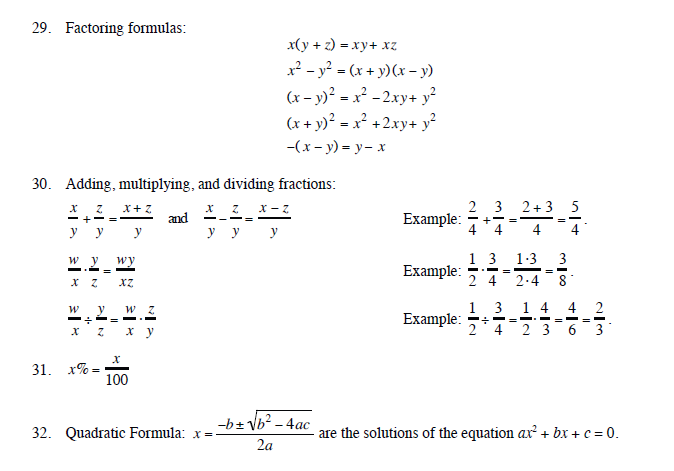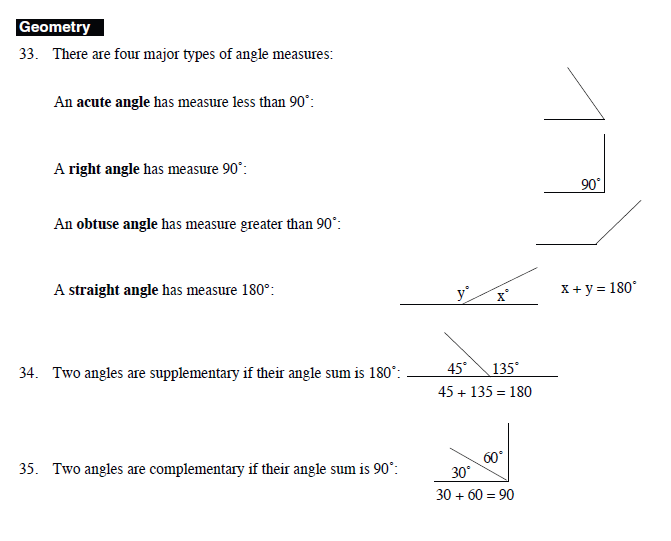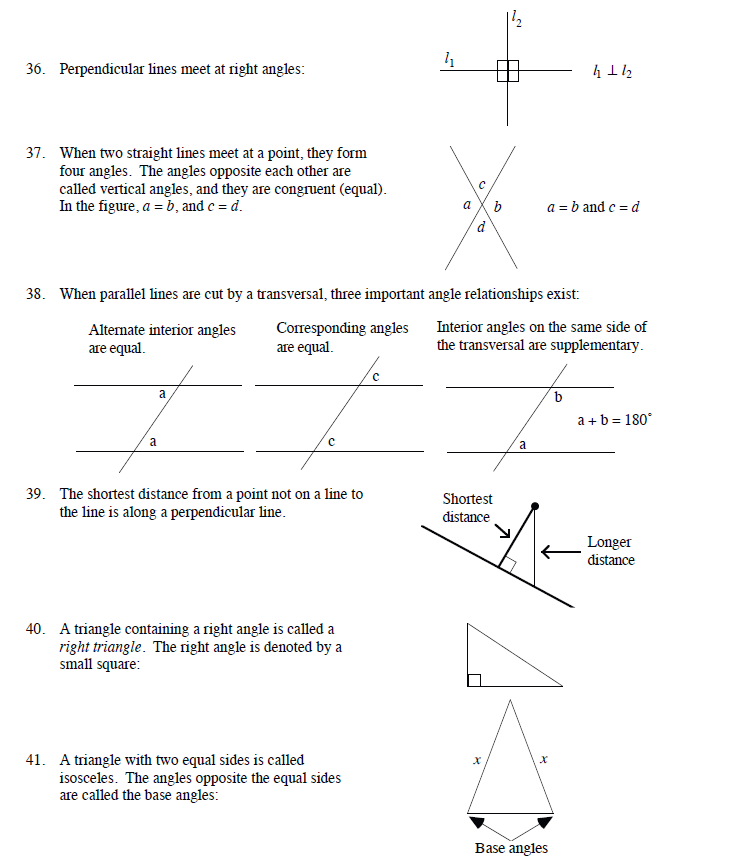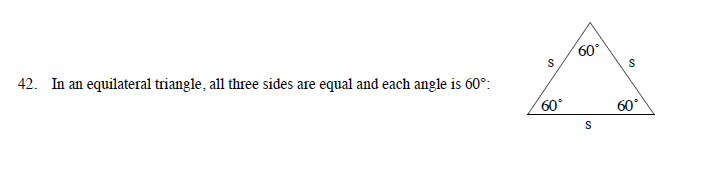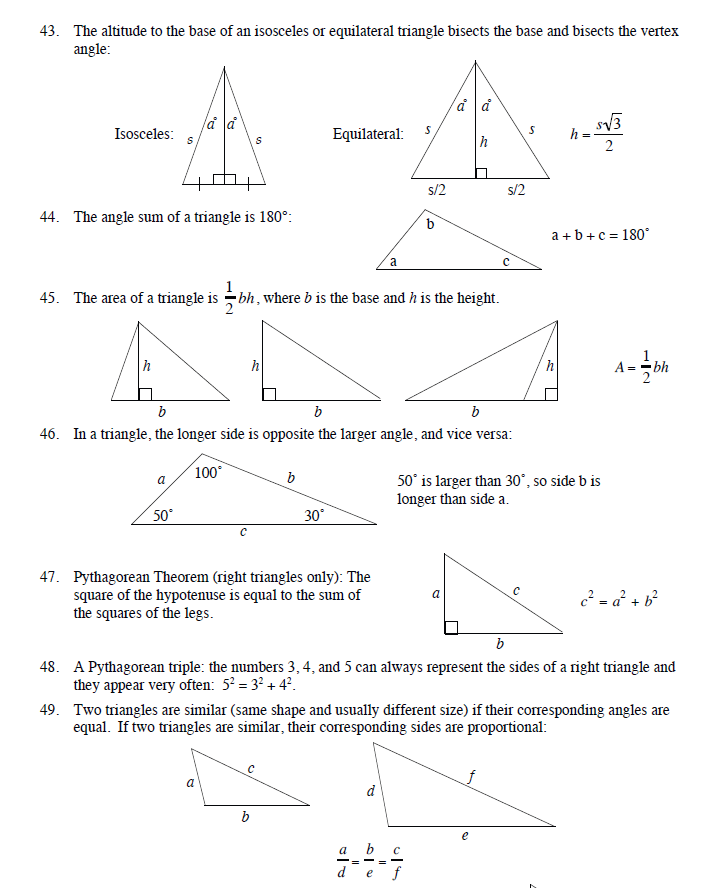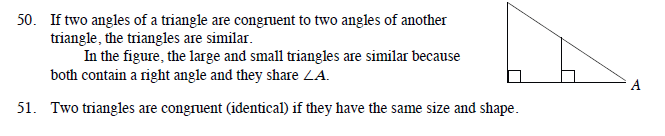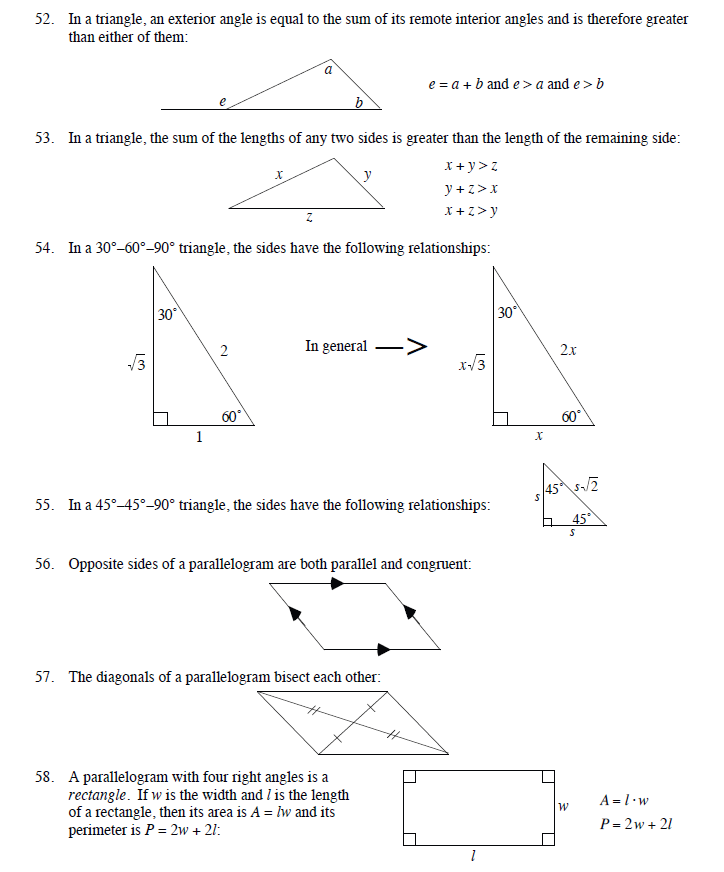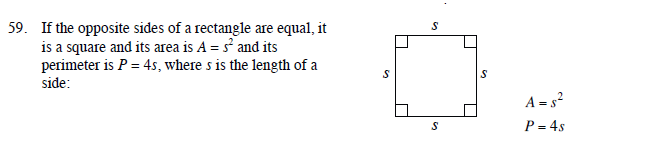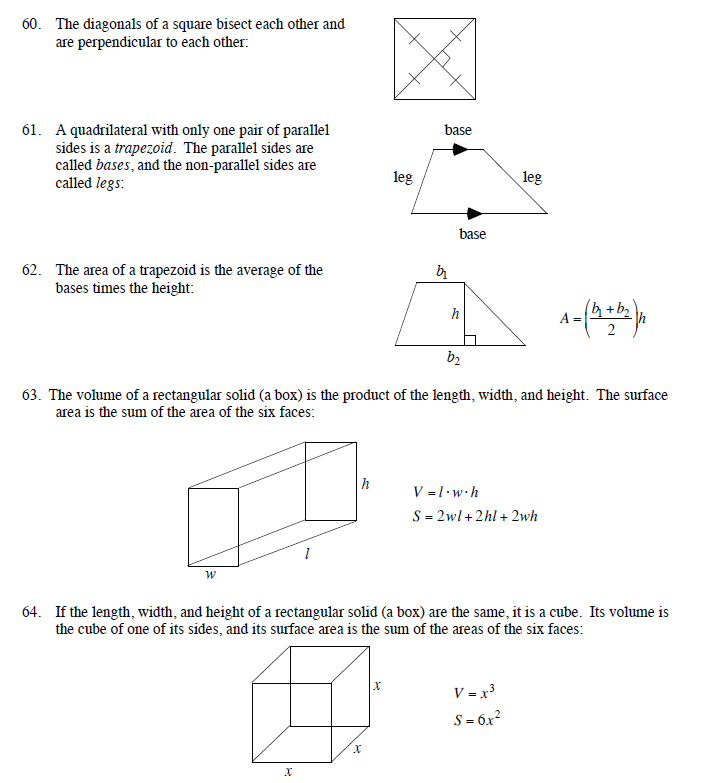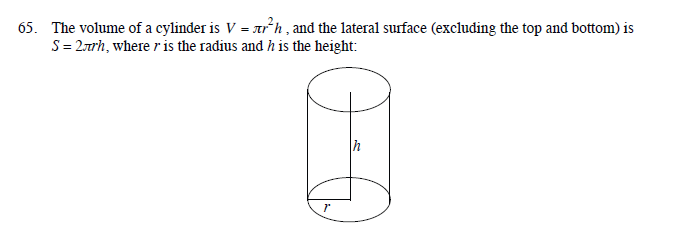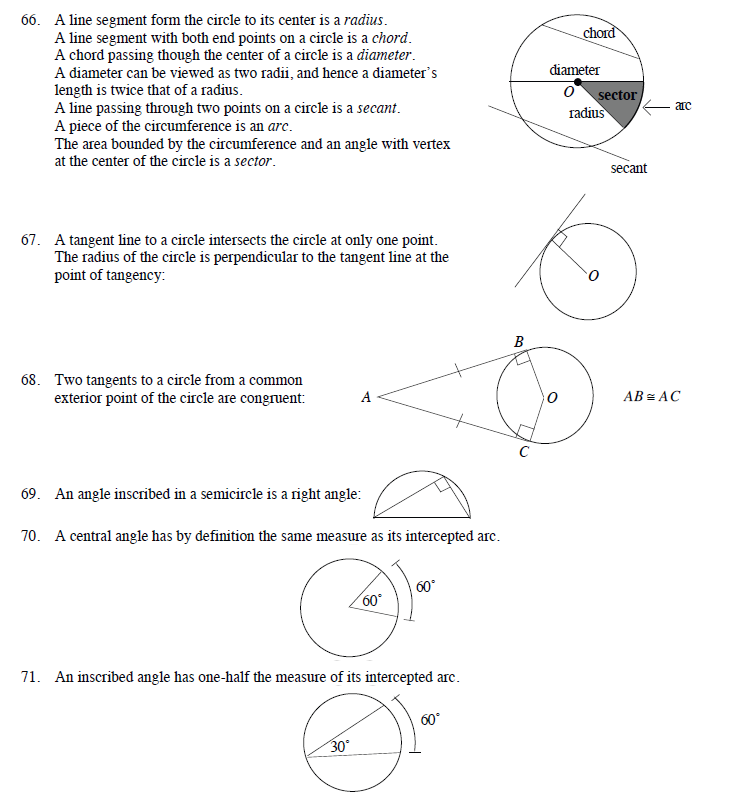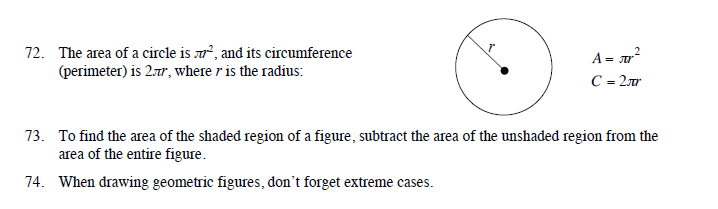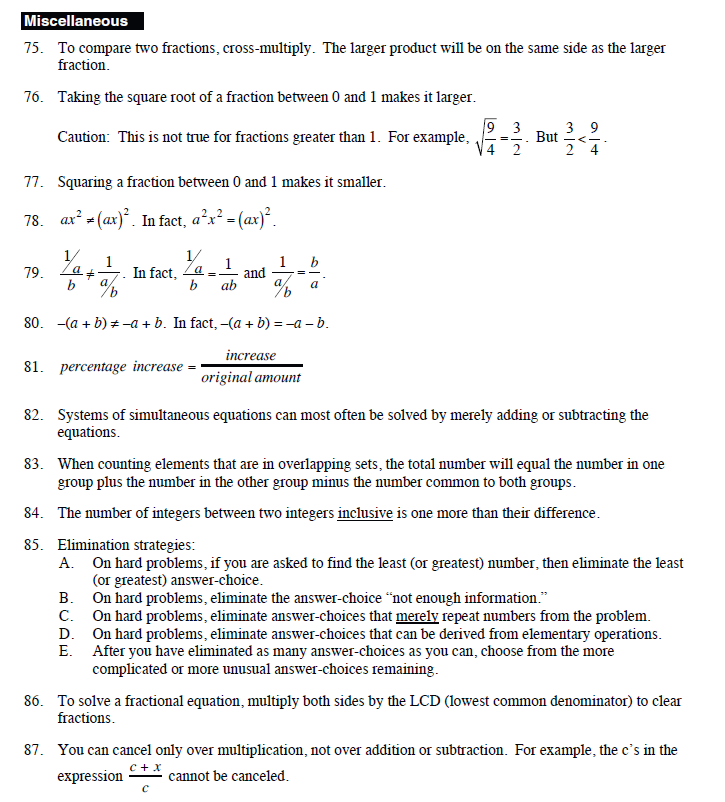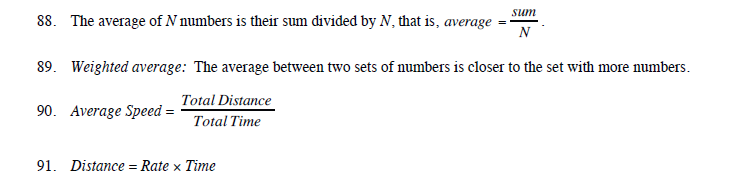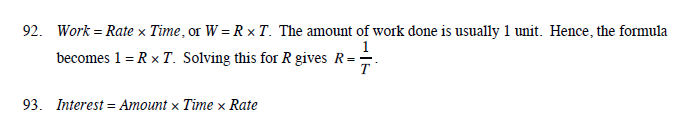Arithmetics Aritmethics.pdf

Algebra Algebra.pdf

Geometry Geometry.pdf

Word problems  Word Problems.pdf

Formulae:

facts-and-formulas-0.pdf

sat-math-vocabulary-0.pdf

Resources:SAT_Math_Essentials.pdf

Tests: SAT Math test.pdf